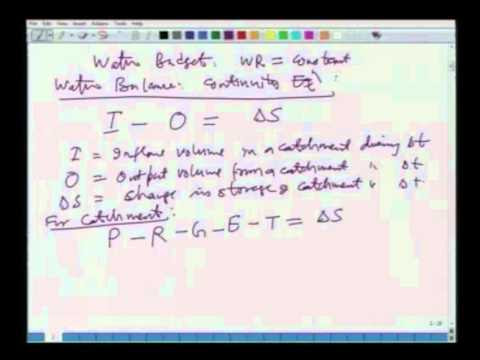This course has been designed to present the principles of advanced hydrology at a postgraduate level.
At the end of the course, a serious student is expected have a thorough understanding of the fundamental mechanisms of various components of hydrologic cycle e.g. atmospheric water, rainfall, infiltration, evaporation, surface flow, sub-surface flow, groundwater flow, and hydrograph analysis;
And learnt the statistical techniques such as statistical properties of a PDF, probability distributions employed in hydrology, fitting probability distributions, testing goodness of fit, frequency analysis, and reliability analysis.

Contents:
Hydrologic cycle, systems concept, hydrologic model classification;
Reynold's Transport Theorem, continuity equation, momentum equation, and energy equation;
Atmospheric hydrology; Hydrologic processes, precipitation, evaporation, surface flow, sub-surface flow, and groundwater flow;
Unit hydrograph, various response functions and their interrelationships; Hydrologic statistics, statistical parameters, fitting a probability distribution, testing goodness of fit, frequency analysis, and reliability analysis.

 Sl. No. Topic No. of Hours 1 INTRODUCTION: Hydrologic cycle, water budget equation, world water quantities, residence time, systems concept, transfer function operators, hydrologic model classification. 03 2 HYDROLOGIC PROCESSES: Reynold's Transport Theorem, continuity equation, momentum equation, energy equation, discrete time continuity. 03 3 ATMOSPHERIC HYDROLOGY: Atmospheric circulation, water vapor, formation of rainfall, types and forms of precipitation, precipitable water, monsoon characteristics in India, rainfall measurement, density and adequacy of rain gauges; Thunderstorm Cell model, IDF relationships, spatial averaging methods of rainfall; Factors affecting evaporation, estimation and measurement of evaporation, energy balance method, aerodynamic method, Priestly-Taylor method, and pan evaporation. 08 4 SUB-SURFACE WATER: Soil moisture, porosity, saturated and unsaturated flow; Richard's equation, infiltration, Horton's Phillip's, and Green Ampt methods, parameter estimation, ponding time concepts. 04 5 SURFACE WATER: Catchment storage concept, Hortonian and saturation overland flow, streamflow hydrographs, base-flow separation. Phi-index, ERH & DRH, algorithm for abstraction using Green-Ampt equation, SCS method, overland and channel flow modeling, time area concepts, and stream networks. 06 6 UNIT HYDROGRAPH: General hydrologic system model, response functions of a linear hydrologic systems and their inter-relationships, convolution equation; definition and limitations of a UH; UH derivation from single and complex storms; UH optimization using regression. matrix, and LP methods; Synthetic unit hydrograph, S-Curve, IUH. 06 7 HYDROLOGIC STATISTICS: Probability concepts, random variables, laws of probability, PDFs & CDFs; Normal and Binomial distributions; Statistical parameters: expected value, variance, skewness, and peakedness; Fitting of a probability distribution, methods of moments and maximum likelihood: Testing the goodness of fit, Chi-square test; Frequency analysis: return period, probability plotting, Extreme value distributions, frequency factors, Log-Pearson distribution, confidence limits. 08 8 GROUNDWATER HYDROLOGY: Occurrence of groundwater, aquifers & their properties, Darcy's law, permeability, transmissibility, stratification, confined groundwater flow, unconfined groundwater flow under Dupit's assumptions; Well hydraulics, steady flow into confined and unconfined wells; Unsteady flow in a confined aquifer. 07